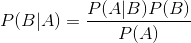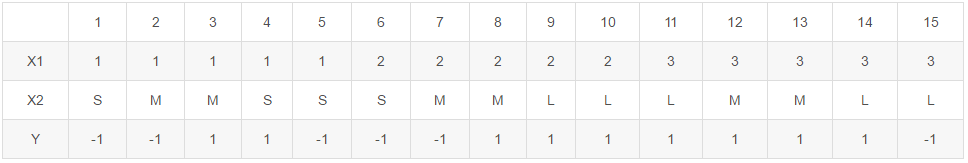朴素贝叶斯算法是流行的十大算法之一，该算法是有监督的学习算法，解决的是分类问题，如客户是否流失、是否值得投资、信用等级评定等多分类问题。该算法的优点在于简单易懂、学习效率高、在某些领域的分类问题中能够与决策树、神经网络相媲美。但由于该算法以自变量之间的独立（条件特征独立）性和连续变量的正态性假设为前提，就会导致算法精度在某种程度上受影响。

# 一、问题的提出

“一所学校里面有 60% 的男生，40% 的女生。男生总是穿长裤，女生则一半穿长裤一半穿裙子。有了这些信息之后我们可以容易地计算“随机选取一个学生，他（她）穿长裤的概率和穿裙子的概率是多大”，这个就是前面说的“正向概率”的计算。然而，假设你走在校园中，迎面走来一个穿长裤的学生（很不幸的是你高度近似，你只看得见他（她）穿的是否长裤，而无法确定他（她）的性别），你能够推断出他（她）是男生的概率是多大吗？”

我们来算一算：假设学校里面人的总数是 U 个。60% 的男生都穿长裤，于是我们得到了 U P(Boy) P(Pants|Boy) 个穿长裤的（男生）（其中 P(Boy) 是男生的概率 = 60%，这里可以简单的理解为男生的比例；P(Pants|Boy) 是条件概率，即在 Boy 这个条件下穿长裤的概率是多大，这里是 100% ，因为所有男生都穿长裤）。40% 的女生里面又有一半（50%）是穿长裤的，于是我们又得到了 U P(Girl) P(Pants|Girl) 个穿长裤的（女生）。加起来一共是 U P(Boy) P(Pants|Boy) + U P(Girl) P(Pants|Girl) 个穿长裤的，其中有 U P(Girl) P(Pants|Girl) 个女生。两者一比就是你要求的答案。

P(Girl|Pants) = P(Girl) P(Pants|Girl) / [P(Boy) P(Pants|Boy) + P(Girl) * P(Pants|Girl)]

注意，如果把上式收缩起来，分母其实就是 P(Pants) ，分子其实就是 P(Pants, Girl) 。而这个比例很自然地就读作：在穿长裤的人（ P(Pants) ）里面有多少（穿长裤）的女孩（ P(Pants, Girl) ）。

P(B|A) = P(A|B) P(B) / [P(A|B) P(B) + P(A|~B) * P(~B) ]

# 二、正式的定义

朴素贝叶斯算法是基于贝叶斯定理与特征条件独立假设的分类方法，然后依据被分类项属于各个类的概率，概率最大者即为所划分的类别。

设输入空间X=（x1,x2,x3…xn），输出类标记为Y=（y1,y2,y3…yn）。x的集合记为X，称为属性集。一般X和Y的关系不确定的，你只能在某种程度上说x有多大可能性属于类y1，比如说x有80%的可能性属于类y1，这时可以把X和Y看做是随机变量，P(Y|X)称为Y的后验概率（posterior probability），与之相对的P(Y)称为Y的先验概率（prior probability），P(X=x|Y=y1)称之为条件概率

通过经验来判断事情发生的概率，比如说“贝叶死”的发病率是万分之一，就是先验概率。再比如南方的梅雨季是 6-7 月，就是通过往年的气候总结出来的经验，这个时候下雨的概率就比其他时间高出很多。

后验概率就是发生结果之后，推测原因的概率。比如说某人查出来了患有“贝叶死”，那么患病的原因可能是 A、B 或 C。患有“贝叶死”是因为原因 A 的概率就是后验概率。它是属于条件概率的一种。

事件 A 在另外一个事件 B 已经发生条件下的发生概率，表示为 P(A|B)，读作“在 B 发生的条件下 A 发生的概率”。比如原因 A 的条件下，患有“贝叶死”的概率，就是条件概率。

简单说来就是：贝叶斯分类算法的理论基于贝叶斯公式：其中P(A|B)称为条件概率，P(B)先验概率，对应P(B|A)为后验概率。朴素贝叶斯分类器基于一个简单的假定，即给定的目标值属性之间是相互独立。贝叶斯公式之所以有用是因为在日常生活中，我们可以很容易得到P(A|B)，而很难得出P(B|A)，但我们更关心P(B|A)，所以就可以根据贝叶斯公式来计算。

# 三、应用举例

如下表所示，训练数据学习一个朴素贝叶斯分类器并确定x=(2,S)T的类标记y。表中x1、x2为特征，取值的集合分别为X1={1,2,3}，X2={S,M,L}，类标记Y={1,-1}P(Y=1)=9/15，P(Y=-1)=6/15

P(X1=1|Y=1)=2/9，P(X1=2|Y=1)=3/9，P(X1=3|Y=1)=4/9

P(X2=S|Y=1)=1/9，P(X2=M|Y=1)=4/9，P(X2=L|Y=1)=4/9

P(X1=1|Y=-1)=3/6，P(X1=2|Y=-1)=2/6，P(X1=3|Y=-1)=1/6

P(X2=S|Y=-1)=3/6，P(X2=M|Y=-1)=2/6，P((X2=L|Y=-1)=1/6

P(Y=1)P(X1=2|Y=1)P(X2=S|Y=1)=(9/15)(3/9)(1/9)=1/45

P(Y=-1)P(X1=2|Y=-1)P(X2=S|Y=-1)=(6/15)(2/6)(1/6)=1/15

# 四、朴素贝叶斯算法的优缺点

1. 朴素贝叶斯模型发源于古典数学理论，有着坚实的数学基础，以及稳定的分类效率；
2. 对大数量训练和查询时具有较高的速度。即使使用超大规模的训练集，针对每个项目通常也只会有相对较少的特征数，并且对项目的训练和分类也仅仅是特征概率的数学运算而已；
3. 对小规模的数据表现很好，能个处理多分类任务，适合增量式训练（即可以实时的对新增的样本进行训练）；
4. 对缺失数据不太敏感，算法也比较简单，常用于文本分类；
5. 朴素贝叶斯对结果解释容易理解。

1. 需要计算先验概率；
2. 分类决策存在错误率；
3. 对输入数据的表达形式很敏感；
4. 由于使用了样本属性独立性的假设，所以如果样本属性有关联时其效果不好。

# 五、应用领域

1. 欺诈检测中使用较多；
2. 一封电子邮件是否是垃圾邮件；
3. 一篇文章应该分到科技、政治，还是体育类；
4. 一段文字表达的是积极的情绪还是消极的情绪；
5. 人脸识别